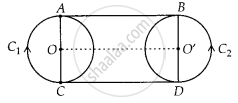# In Question 5 above, if radii of the two circles are equal, prove that AB = CD. - Mathematics

Sum

In Question 5 above, if radii of the two circles are equal, prove that AB = CD.

#### SolutionJoin OO’

Since, OA = O’B  ......[Given]

Also, ∠OAB = ∠O’BA = 90°   .......[Tangent at any point of a circle is perpendicular to the radius at the point of contact]

Since, perpendicular distance between two straight lines at two different points is same.

⇒ AB is parallel to OO’

Similarly, CD is parallel to OO’

⇒AB ॥ CD

Also, ∠OAB = ∠OCD = ∠O’BA = ∠O’DC = 90°

⇒ ABCD is a rectangle.

Hence, AB = CD.

Concept: Concept of Circle - Centre, Radius, Diameter, Arc, Sector, Chord, Segment, Semicircle, Circumference, Interior and Exterior, Concentric Circles
Is there an error in this question or solution?

#### APPEARS IN

NCERT Mathematics Exemplar Class 10
Chapter 9 Circles
Exercise 9.3 | Q 6 | Page 107
Share